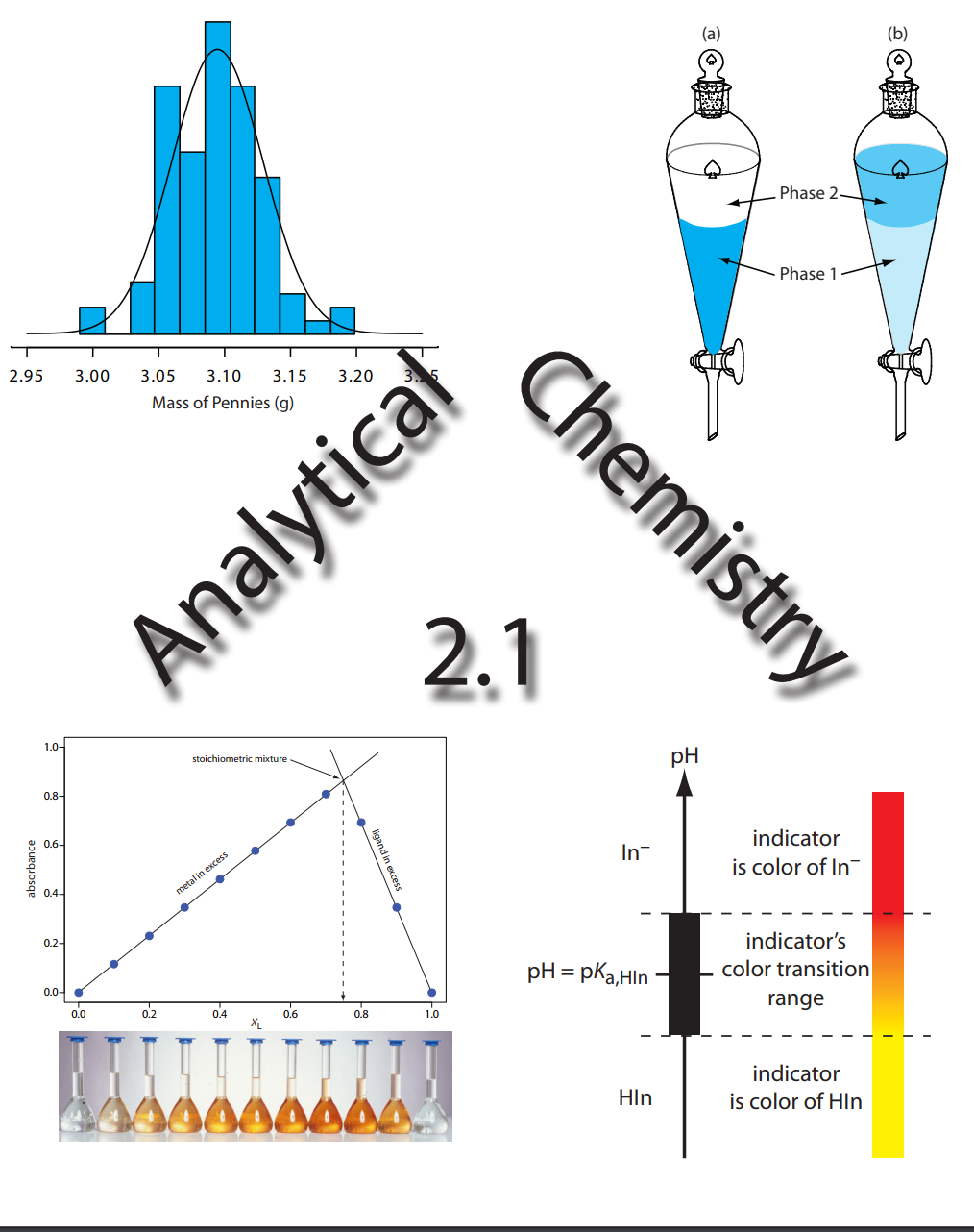# Analytical Chemistry 2.1

(12 reviews)David Harvey, DePauw University

Publisher: David T. Harvey

Language: English

## Conditions of UseAttribution-NonCommercial-ShareAlike
CC BY-NC-SA

## Reviews

• Chapter 1: Introduction to Analytical Chemistry
• Chapter 2: Basic Tools of Analytical Chemistry
• Chapter 3: The Vocabulary of Analytical Chemistry
• Chapter 4: Evaluating Analytical Data
• Chapter 5: Standardizing Analytical Methods
• Chapter 6: Equilibrium Chemistry
• Chapter 7: Obtaining and Preparing Samples for Analysis
• Chapter 8: Gravimetric Methods
• Chapter 9: Titrimetric Methods
• Chapter 10: Spectroscopic Methods
• Chapter 11: Electrochemical Methods
• Chapter 12: Chromatographic & Electrophoretic Methods
• Chapter 13: Kinetic Methods
• Chapter 14: Developing a Standard Method
• Chapter 15: Quality Assurance
• Appendices

## Ancillary Material

• David T. Harvey

As currently taught in the United States, introductory courses in analytical chemistry emphasize quantitative (and sometimes qualitative) methods of analysis along with a heavy dose of equilibrium chemistry. Analytical chemistry, however, is much more than a collection of analytical methods and an understanding of equilibrium chemistry; it is an approach to solving chemical problems. Although equilibrium chemistry and analytical methods are important, their coverage should not come at the expense of other equally important topics.

The introductory course in analytical chemistry is the ideal place in the undergraduate chemistry curriculum for exploring topics such as experimental design, sampling, calibration strategies, standardization, optimization, statistics, and the validation of experimental results. Analytical methods come and go, but best practices for designing and validating analytical methods are universal. Because chemistry is an experimental science it is essential that all chemistry students understand the importance of making good measurements.

My goal in preparing this textbook is to find a more appropriate balance between theory and practice, between “classical” and “modern” analytical methods, between analyzing samples and collecting samples and preparing them for analysis, and between analytical methods and data analysis. There is more material here than anyone can cover in one semester; it is my hope that the diversity of topics will meet the needs of different instructors, while, perhaps, suggesting some new topics to cover.